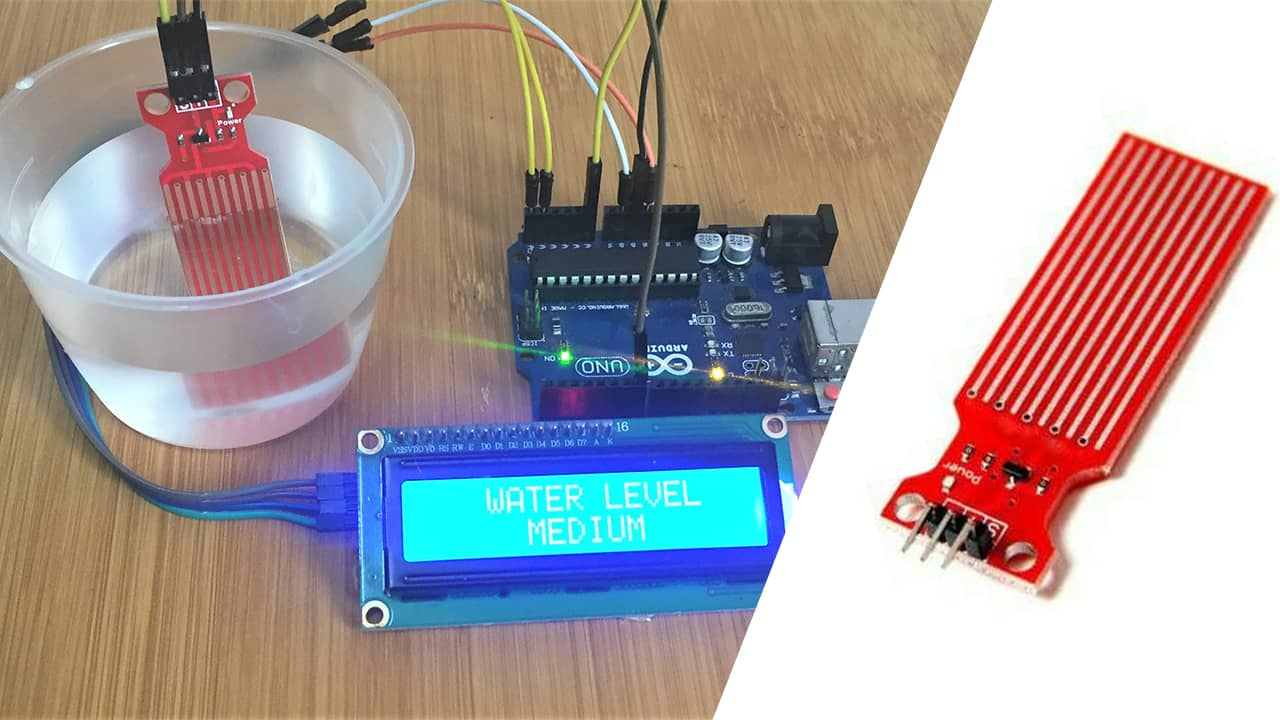# Water Level Sensor with Arduino.Water level sensors are used in a number of areas involving monitoring the levels and flow of water like in tanks for irrigation systems, swimming pools and fish ponds. In this tutorial I will show how we can interface this kind of sensor with Arduino.

## How does the water level sensor work?

This sensor actually responds to conductivity of the water where it is immersed. The conductivity of water depends on the amount of impurities in the water therefore pure water is not conductive.

The sensor has ten copper traces, five of which are power traces and five are sense traces. These traces are not connected but are bridged by water when submerged and act as a variable resistor whose resistance varies according to the water level.

This resistance is inversely proportional to the height of the water, that is, the more water the sensor is immersed in, results in better conductivity and will result in a lower resistance and vice versa. The sensor produces an output voltage according to the resistance and this voltage is interpreted by a microcontroller to determine the water level.

## Connecting the water level sensor to Arduino.

Before using the water level sensor , we need to calibrate it so that we can get threshold values for a given sample of water since the conductivity of water varies.The ‘-‘ is connected to Arduino GND, ‘+’ is connected to 5V and ‘S’ is connected to an analog pin of the Arduino as shown in the schematic below.

The code sketch below is then uploaded to the Arduino and open the serial monitor.

## Code for calibration of the water level sensor with Arduino.

``````const int analogInPin = A0;
int sensorValue = 0;

void setup() {
Serial.begin(9600);
}

void loop() {

Serial.print("Sensor = " );
Serial.println(sensorValue);

delay(1000);
}
``````

The sensor is slowly submerged into a container with water and the various values are observed on the serial monitor. Values should begin from zero when not submerged and then go on increasing as more of the sensor is submerged in the water.

From the values on the serial monitor we can select values to use as our lower and upper thresholds. For example from the above photo we can use 310 and 510 as our lower and upper thresholds respectively.

## Making a simple water level monitoring system.

We can now be able to use the sensor to detect the level of water in a given container for example the set up below shows how the sensor can be connected to an I2C lcd so that we can be able to display the water level on the lcd depending on the thresholds set in the corresponding code.

You can review how to use the I2C LCD with Arduino from a previous post using the link below

• How to interface I2C LCD with Arduino
• From the schematic above you can observe that the power terminal of the sensor is not connected to the 5V pin of the Arduino as before but rather to a digital pin . This is done to reduce the rate of corrosion of the copper when they are submerged in water and powered for a long time.

In this case we only power the traces when we are taking a reading from the sensor by making the digital pin high. This will increase the life span of the sensor.

### Code for the water level monitoring system using Arduino.

The major issue in the code below is setting the threshold values depending on how you did the calibration.

``````#include <Wire.h>
#include <LiquidCrystal_I2C.h>

LiquidCrystal_I2C lcd(0x27, 2, 1, 0, 4, 5, 6, 7, 3, POSITIVE);

int lowerThreshold = 310;
int upperThreshold = 510;

// Sensor pins
#define sensorPower 7
#define sensorPin A0
int val = 0; // Value for storing water level

void setup() {
Serial.begin(9600);
lcd.begin(16,2);
lcd.backlight();
pinMode(sensorPower, OUTPUT);
digitalWrite(sensorPower, LOW);
}

void loop() {

if (level == 0) {
Serial.println("Water Level: Empty");
lcd.setCursor(0,0);
lcd.print("   WATER LEVEL ");
lcd.setCursor(0,1);
lcd.print("     EMPTY      ");
}
else if (level > 0 && level <= lowerThreshold) {
Serial.println("Water Level: Low");
lcd.setCursor(0,0);
lcd.print("   WATER LEVEL ");
lcd.setCursor(0,1);
lcd.print("      LOW       ");

}
else if (level > lowerThreshold && level <= upperThreshold) {
Serial.println("Water Level: Medium");
lcd.setCursor(0,0);
lcd.print("   WATER LEVEL ");
lcd.setCursor(0,1);
lcd.print("     MEDIUM     ");
}
else if (level > upperThreshold) {
Serial.println("Water Level: High");
lcd.setCursor(0,0);
lcd.print("   WATER LEVEL ");
lcd.setCursor(0,1);
lcd.print("     FULL       ");
}
delay(1000);
}

//This is a function used to get the reading